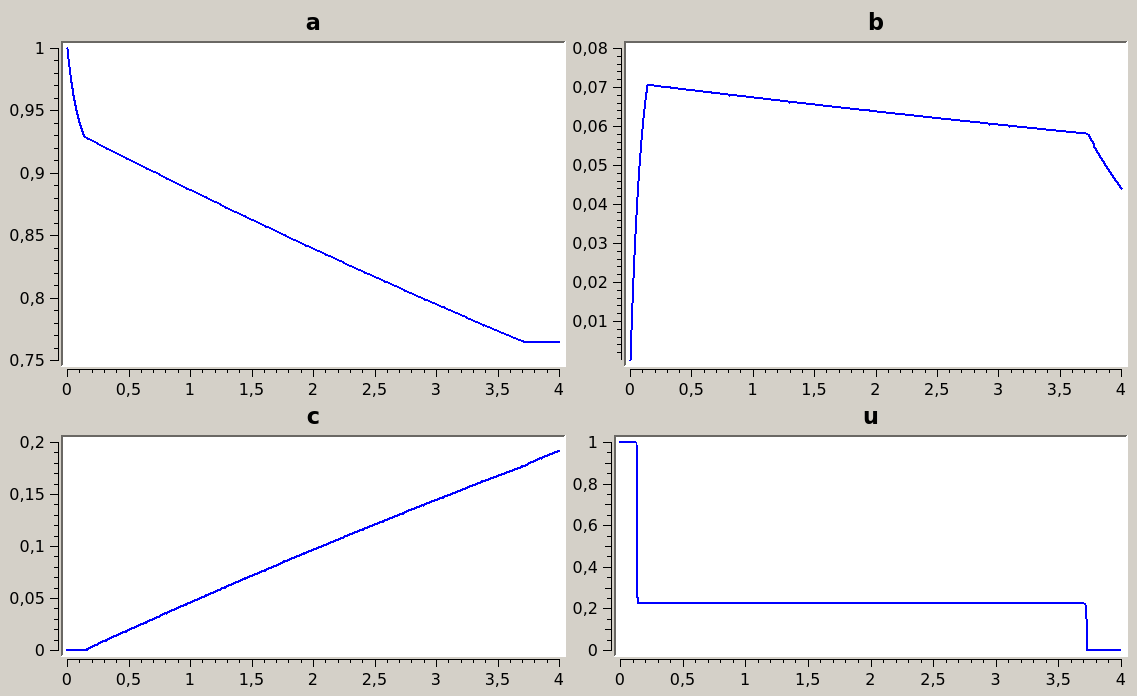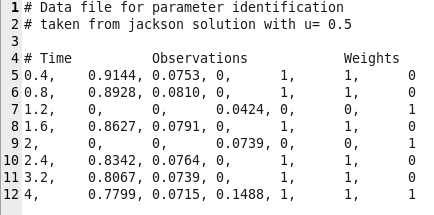# Jackson problem – Parameter identificationConsider the Jackson model (,) of chemical reactions$Latex formula$

The first reaction is reversible, converting A to B and vice-versa, and the second one is one-sided, converting B to C. Here the control$Latex formula$ is the fraction of catalyst which sets the balance between the reactions 1 and 2, and we want to maximize the production of C. The feed is assumed to consist of pure substance A. Noting a, b, c the mole fractions of A, B, C and k1, k2, k3 the velocity constants of chemical reactions, the optimal control problem is written as$Latex formula$

Remark: note that since a(t) + b(t) + c(t) is an invariant, we could eliminate one of the state variable.

The Hamiltonian being linear in the control, we expect a solution whose optimal control is a sequence of bang and/or singular arcs. We show below the solution obtained for k1=k3=1, k2=10, and T = 4. In this case the control has one singular arc, with a bang (1) – singular – bang(0) structure.

Numerical simulations: problem jackson
Discretization: Lobatto (6th order) with 500 steps.Parameter identification
Consider now the same optimization problem where k1, k2 and k3 are unknown parameters. We want to identify these parameters based on some observations of the concentrations a(t), b(t) and c(t) from an experiment with a known control. Here we will replace the experiment with a simulation, taking for instance the constant control u(t) = 0.5. For this simulation we also set the parameters to the values k1 = k3 = 1, k2 = 10. Obviously we expect the identification to give values close to these. We take some sample values from the simulation and add some small noise to reflect the errors in measurements, see . In this case we made the assumption that a, b and c were measured at different time steps, hence the use of weights equal to 0 or 1 in the observation file.Figure 1: Comma-separated observation data

Then we perform the identification on k1,k2,k3, using theses values as observation data, with the least square method featured in Bocop. The results of the identification are very close to the original values, with k1=0.997614, k2=9.97377 and k3=1.00095.

References
 R.Jackson. Optimal use of mixed catalysts for two successive reactions. J.Optimization Theory applications, 2(1):27-39, 1968.

 L.T. Biegler. Nonlinear  programming, volume 10 of MOS-SIAM Series on Optimization. Society for Industrial and Applied Mathematics (SIAM), Philadelphia, PA, 2010. Concepts, algorithms, and applications to chemical processes.